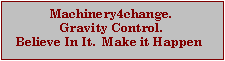high B.5,B.6,B.7,B.4,B.3 'Set Enable Pins High  4511 Enable is Low
'B.3 Blank Enable, leading zero hundreds blanked

for b9 = 1 to 3 'Lamp Test, startup flash all segments 3 times x 0.5 sec
low B.4         'Test LEDs
pause 250
high B.4
pause 250
next b9
symbol dlay = b8
dlay = 10
symbol num = b7
b9 = 1

do while b9 = 1

main:

count B.1,2000,w0 'w0 = b1:b0 counts pulses 0.2 meters apart for 2 seconds.
'this gives 10 times the number for 1 m/sec allowing 1
w0 = w0*2                 'dec place on panel meter. This also allows a finer
'debug                  'resolution for the codeout setpoints.

bintoascii w0,b2,b3,b4,b5,b6           'b4 = hundreds, b5 = tens, b6 = units
b2 = b2 - 48                           'b2 = 10 thous b3 = thous not used here
b3 = b3 - 48                           'but variables reserved
b4 = b4 - 48
b5 = b5 - 48
b6 = b6 - 48

'makedigits: 'using 12345 This may also save some variables.
'remain = counting // 10000  'gives remainder 2345
'digit  = counting - remain /10000
'b15 = digit
'poke 86,digit       'tenthou

'remain2 = remain // 1000  'remain2 = remain // 1000 gives remainder 345
'digit  = remain - remain2 /1000
'b16 = digit
'poke 87,digit '      thou

'remain = remain2 // 100  'gives remainder 345
'digit  = remain2 - remain /100
'b17 = digit
'poke 88,digit 'hundreds
'remain2 = remain // 10  'gives remainder 345
'digit  = remain - remain2 /10
'b18 = digit
'poke 89, digit 'tens
'b19 = remain2
'poke 90, remain2 'units

'digit = 0
'peek 86,digit
return
num = b4
if num < 1 then low B.3 else high B.3 endif
gosub selectno
low B.7
pause dlay
high B.7

num = b5
gosub selectno
low B.6
pause dlay
high B.6

num = b6
gosub selectno
low B.5
pause dlay
high B.5

gosub codeout

loop
'goto main

selectno:
if num = 0 then
' switch outputs 7,6,1,0 low for numeral 0
low C.7,C.6,C.1,C.0
endif
if num = 1 then
low C.1,C.0,C.7                                 ' switch output 6 high for       numeral 1
high C.6
endif
if num = 2 then
low C.0,C.6,C.7                                 ' switch output 1 high for       numeral 2
high C.1
endif
if num = 3 then
low C.0,C.7                                 ' switch output 1 and 6 high for numeral 3
high C.1,C.6
endif
if num = 4 then
low C.1,C.6,C.7                                 ' switch output 0 high for       numeral 4
high C.0
endif
if num = 5 then
low C.1,C.7                                 ' switch output 0 and 6 high for numeral 5
high C.0,C.6
endif
if num = 6 then
low C.6,C.7                                 ' switch output 0 and 1 high for numeral 6
high C.0,C.1
endif
if num = 7 then
low C.7                                 ' switch output 0,1,6, high for  numeral 7
high C.0,C.1,C.6
endif
if num = 8 then
low C.0,C.1,C.6                                 ' switch output 7 high for       numeral 8
high C.7
endif
if num = 9 then
low C.0,C.1                                 ' switch output 6,7,high for     numeral 9
high C.6,C.7
endif
'output C.6 = Data A on 4511 IC
'output C.1 = Data B on 4511 IC
'output C.0 = Data C on 4511 IC
'output C.7 = Data D on 4511 IC
return                          'output B.7 = Enable Hundreds Display
'output B.6 = Enable Tens Display
'output B.5 = Enable Units Display

codeout:  'codes sent to guide wheels picaxe40X2 micro

w13 = w0   'All numbers in w13 are 10x eg 28 = 2.8 m/s

if w13 = 0 then 'code 7  111  All motorized guide wheels must be extended.Activate stabilizers and
high B.2       'allow rotor to start accelerating to code 6
high B.0
high C.2       'If in shutdown mode, deactivate stabilizers
end if

if w13 > 28 and w13 < 36 then 'code 6  110 Activate motorized guide wheels
high B.2                  ' to retract then accel to code 5
high B.0
low  C.2
end if

if w13 > 38 and w13 < 46 then 'code 5  101 All motorized guide wheels should now be
high B.2                  'retracted. Rotor should be turning unsuported.Deactivate
low  B.0                  'stabilizers.
high C.2                  'If Shutdown Mode Extend all motorized guide wheels.
end if                    'May need a delay here.

if w13 > 58 AND w13 < 6 then 'code 4
high B.2
low  B.0
low  C.2
end if

if w13 > 98 AND w13 < 110 then 'code 3  010 'control rotor upper speed limit
low B.2    'power will shut off at 9.0mps, rotor will cycle between 9.0 and 8.0mps
high  B.0  'code 3 appears as speed drops to 8.0mps
low  C.2
end if

if w13 > 82 AND w13 < 92 then 'code 4  011 'control rotor lower speed limit
low  B.2        'code changes at 8.0mps, first pass power is already on, accel until
high B.0        'code 2 where power shuts off at code 2. Subsequent passes
high C.2        'will power on as speed drops to 8.0mps and code changes back to 3
end if

if w13 > 130  then 'code 2 001 automatic shutdown. if rotor speed exceeds limit the
low  B.2         'shutdown proceedure initiates. Manual power reset required to
low  B.0         'restart rotor. Shutdown bypasses codes 2 & 3
high C.2
end if

return